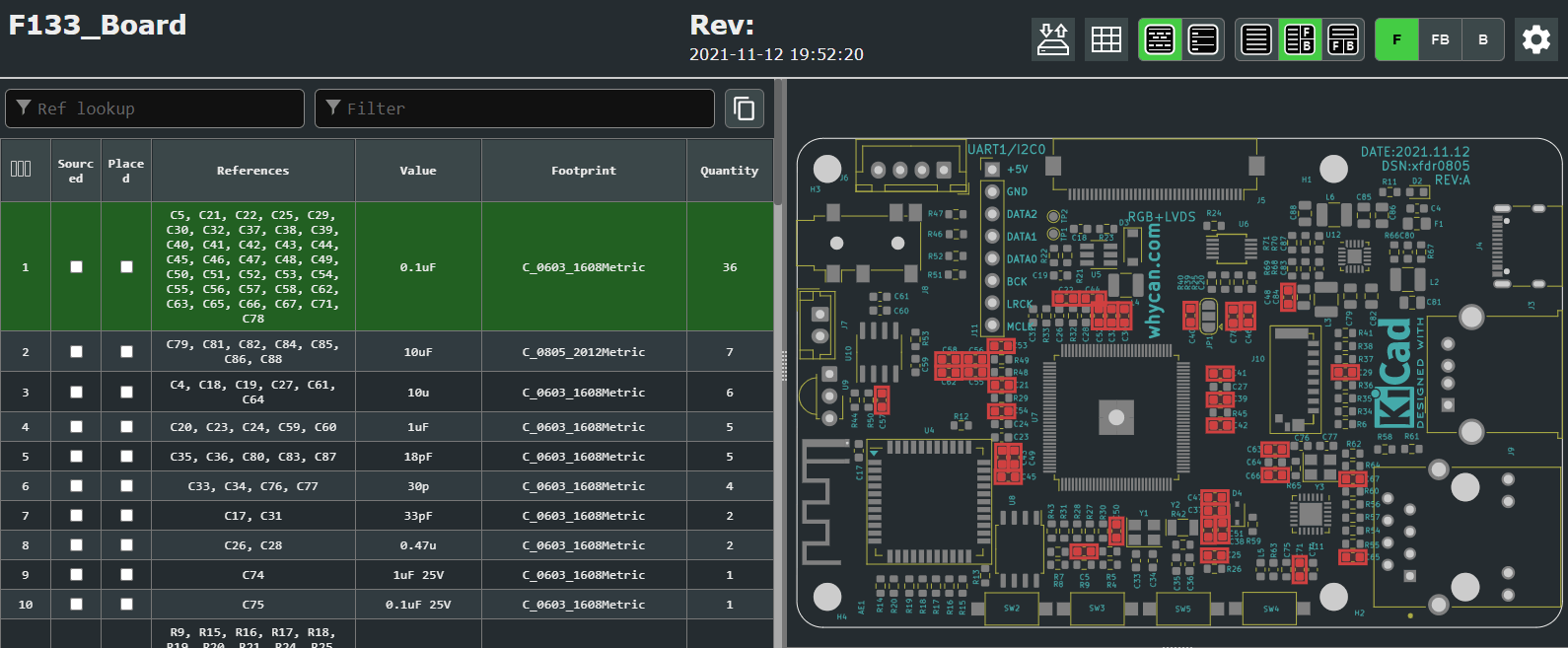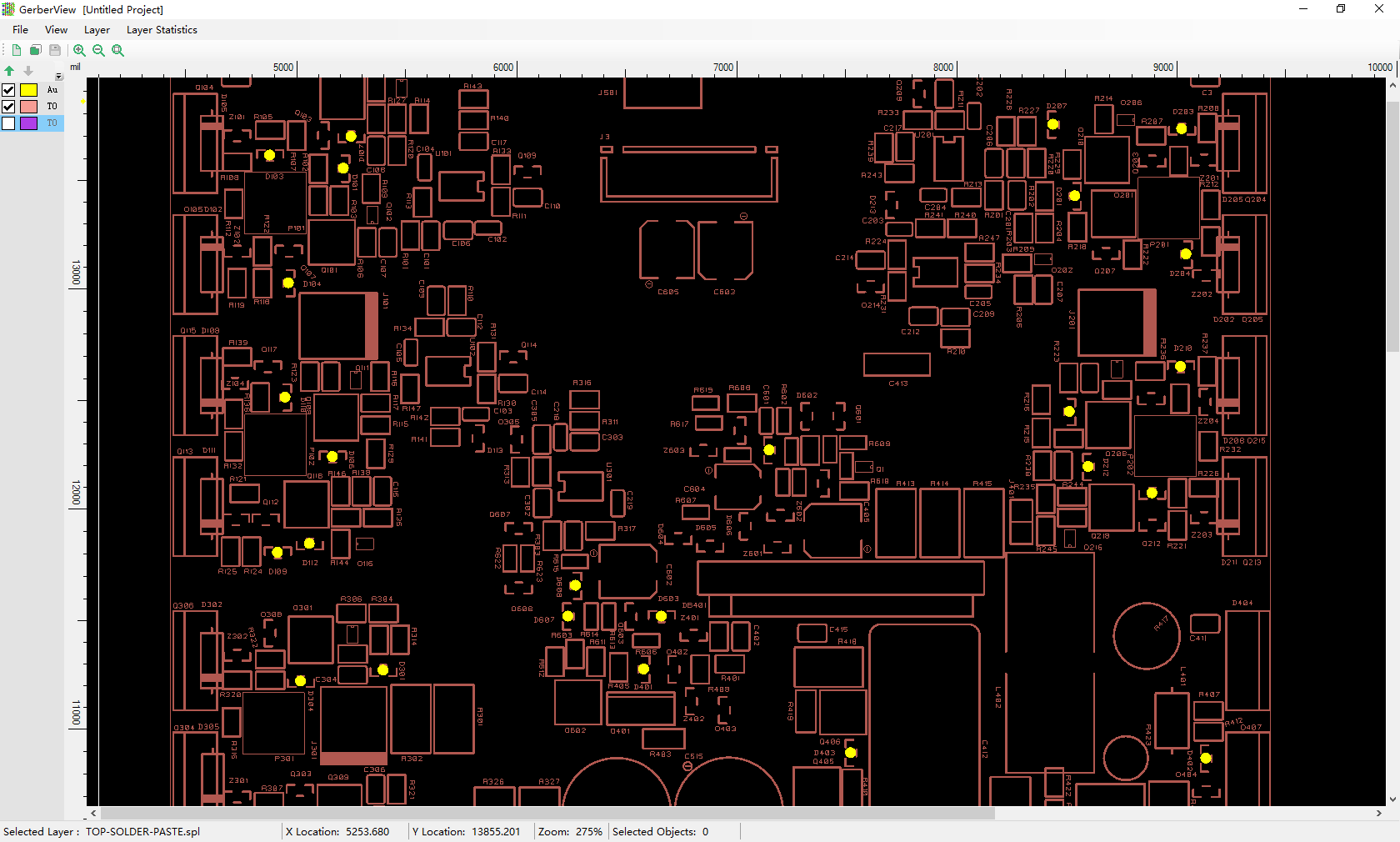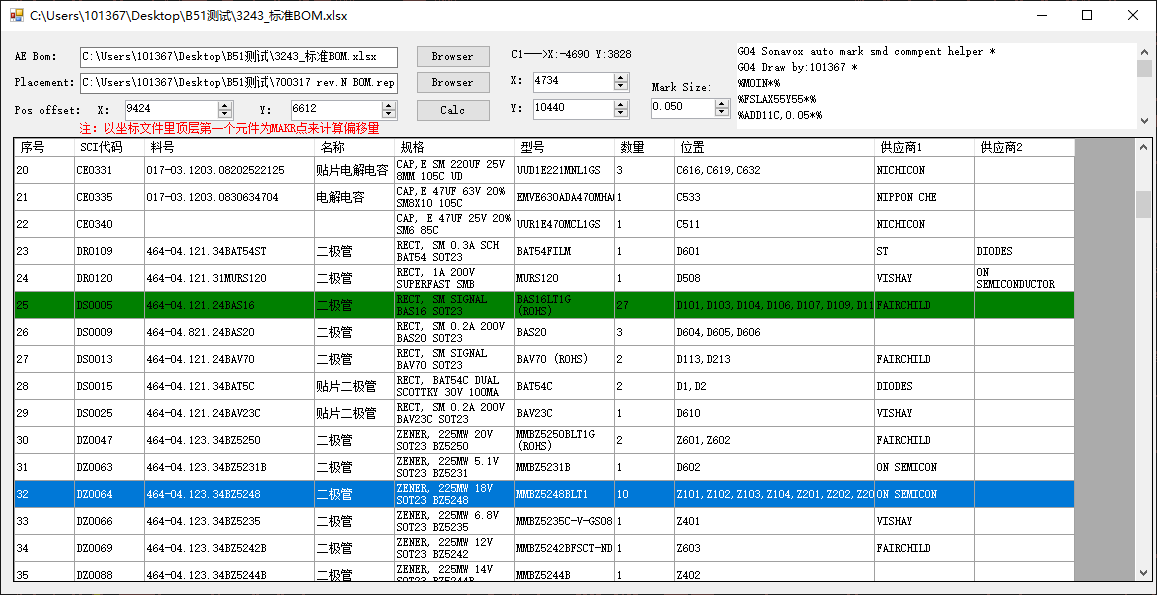# WhyCan Forum(哇酷开发者社区)

## 楼主 # 2021-12-10 20:46:44 分享评论

xfdr0805### 分享一个-手工贴片助手-C#开发

##### 灵感来源##### Gerber文件解析

Gerber文件要是自己写，还是比较复杂的，网上已有很多开源的Gerber文件解析的库,就不重复造轮子了，这里使用了miltonneal54大神开源的GerberVS库，我测试了一下，已经相当完善了，测试了一些产品的gerber 基本没问题。
Gerber解析库
https://github.com/miltonneal54/GerberVS
Gerber规范
gerber_rs274xrevd_e.pdf

##### BOM表解析

BOM是excel格式的，这里使用开源的NPOI进行BOM表的读取，还是比较简单的

``````workBook = new XSSFWorkbook(tbx_bom.Text);
ISheet sheet = workBook.GetSheetAt(0);//"PCB 部分"
//计算总行数
int lastNum = sheet.LastRowNum;
Console.WriteLine("总行数:" + lastNum);
//从第1行开始
//Console.WriteLine(sheet.GetRow(3).GetCell(1).StringCellValue);
dataGridView1.Rows.Clear();
for (int i = 2; i < lastNum; i++)
{
if (sheet.GetRow(i) != null)
{
//Console.WriteLine(i + " ---> " + sheet.GetRow(i).Count());
for (int j = 0; j < 10; j++)
{
if (sheet.GetRow(i).GetCell(j) != null)
{
if (sheet.GetRow(i).Cells.Count > 10)
{
//dataGridView1.Rows[i].Cells[j].Value = sheet.GetRow(i).GetCell(j).StringCellValue;
switch (sheet.GetRow(i).GetCell(j).CellType)
{
case CellType.Numeric:
//Console.WriteLine(j + " ---> " + sheet.GetRow(i).GetCell(j).NumericCellValue);
dataGridView1.Rows[i - 2].Cells[j].Value = sheet.GetRow(i).GetCell(j).NumericCellValue;
break;
case CellType.String:
//Console.WriteLine(j + " ---> " + sheet.GetRow(i).GetCell(j).StringCellValue);
dataGridView1.Rows[i - 2].Cells[j].Value = sheet.GetRow(i).GetCell(j).StringCellValue;

break;

}

}
else
{
Console.WriteLine(i + " ---> cells < 10");
}

}

}
}

}
//dataGridView1.AutoResizeColumns();
dataGridView1.AutoResizeRows();``````
##### 坐标文件解析

``````StreamReader sr = File.OpenText(tbx_rep.Text);
pst = new List<Placement>();
while ((temp = sr.ReadLine()) != null)
{

if (temp.Contains("Total Number of SMD Components on Top"))
{
string s = temp.Remove(0, temp.IndexOf(':') + 1);
line = int.Parse(s);
for (int i = 0; i < line; i++)
{

//坐标文件格式 座位号 X Y 长度都为10 角度为7

string str = temp.Substring(0, 10).Trim();
string x = temp.Substring(10, 10).Trim();
string y = temp.Substring(20, 10).Trim();
string r = temp.Substring(30, 7).Trim();
if (i == 0)
{
label_first_smd.Text = string.Format("{0}--->X:{1} Y:{2}", str, x, y);
}
Placement p = new Placement();
p.Symbol = str;
p.X_pos = x;
p.Y_pos = y;
p.Rotation = r;
}
}
}``````
##### 在图形中渲染位置

``````//获取座位号
Console.WriteLine(dataGridView1.Rows[e.RowIndex].Cells.Value);
string location = (string)dataGridView1.Rows[e.RowIndex].Cells.Value;
string[] loc;
StringBuilder sb = new StringBuilder();
sb.Append("G04 Sonavox auto mark smd commpent helper *\r\n");
sb.Append("G04 Draw by:101367 *\n");
sb.Append("%MOIN*%\n");//单位选择
sb.Append("%FSLAX55Y55*%\n");//坐标系选择
sb.Append("\nG54D11*\n");//选择自定义光圈
if (location != "")
{
loc = location.Split(',');
bool find = false;
foreach (string s in loc)
{
find = false;
foreach (Placement p in pst)
{

if (s == p.Symbol)
{
find = true;
Int32 xpos = Int32.Parse(p.X_pos) + (Int32)numericUpDown_x.Value;
Int32 ypos = Int32.Parse(p.Y_pos) + (Int32)numericUpDown_y.Value;
xpos = xpos * 100;
ypos = ypos * 100;
sb.Append(string.Format("X{0}Y{1}D03*\n", xpos, ypos));//标识位置
Console.WriteLine(string.Format("Top SMD:{0}--->X:{1} Y:{2} R:{3}", s, p.X_pos, p.Y_pos, p.Rotation));
//break;
continue;//跳过剩下的代码，继续执行循环
}

}
if (find == false)
{
foreach (Placement p in psb)
{
if (s == p.Symbol)
{
find = true;
Int32 xpos = Int32.Parse(p.X_pos) + (Int32)numericUpDown_x.Value;
Int32 ypos = Int32.Parse(p.Y_pos) + (Int32)numericUpDown_y.Value;
xpos = xpos * 100;
ypos = ypos * 100;
sb.Append(string.Format("X{0,0.00}Y{1,0.00}D03*\n", xpos, ypos));//标识位置

Console.WriteLine(string.Format("Bottom SMD:{0}--->X:{1} Y:{2} R:{3}", s, p.X_pos, p.Y_pos, p.Rotation));
continue;//跳过剩下的代码，继续执行循环
}

}
}

if (find == false)
{
MessageBox.Show(s + "的坐标未找到，请确认！！！\r\n可能是座位号不正确或者在MI部分");
return;
}
}
sb.Append("M02*\n");
richTextBox1.Text = sb.ToString();
StreamWriter sw = new StreamWriter(@"Auto-Generate-Gerber.gbx");
sw.Write(sb.ToString());
sw.Flush();
sw.Close();
}``````
##### 软件界面GerberVS.7z

yangshishu

david

Quotation

Gentlepig

jack123321

Timaker

xiaotiangg

xpulabs

haistart

• 不通过:其他

xfdr0805

john78

xfdr0805

xfdr0805

john78 说：

lxj1997

• 不通过:其他